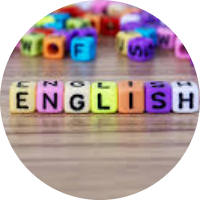# Mathematics and its Applications

This set of MCQs helps you brush up on important math topics and prepare you to dive into skill practice.

Start Quiz

Per, Ragnar, and Lars live in the same neighborhood. They have found out that the straight line distance from Per's house to Ragnar's house is 250 m, and from Ragnar's house to Lars' house is 300 m. Which of the following is true about the distance between Per's house and Lars' house?

The distance is between 0 m and 550 m.

The distance is precisely 550 m.

The distance is between 250 m and 300 m.

The distance is between 50 m and 550 m.

All numbers can be written in base three, in a way similar to base ten. The difference is that in base three, only the digits 0, 1, and 2 can be used. The numbers which we write in base ten as 1, 2, 3, 4, 5, and so on, are written in base three as 1, 2, 10, 11, 12, and so forth. What number in base ten corresponds to the number 1021 in base three?

22

42

40

41

Let us call a positive integer "lucky" if its digits can be divided into two groups so that the sum of the digits in each group is the same. For example, 34175 is lucky because 3 + 7 = 4 + 1 + 5. Find the smallest 4- digit lucky number, whose neighbor is also a lucky number (i.e. the integer next to it is a lucky number as well).

1449

1445

1450

1451

There are eight positive integers in a row. Starting from the third, each is the sum of the two numbers before it. If the eighth number is 2017, what is the largest possible value of the first one?

11

10

15

20

A box contains a total of 400 tickets in five different colors: blue, green, red, yellow, and orange. The ratio of blue to green to red tickets is 1: 2: 4. The ratio of green to yellow to orange tickets is 1: 3: 6. What is the smallest number of tickets that must be drawn to ensure that at least 50 tickets of one color have been selected?

196

194

195

199

If the radius of a hemisphere is 2r, find its volume.

16/3* πr^3.

4/3* πr^3.

2/3* πr^3.

16/3* πr^3.

A rectangular playground has length and width in the ratio of 3:4. If the area of the playground is 7500 sq. m., then the length of a diagonal path through the playground is,

123

125

130

225

The difference between the two natural numbers is 59. If the larger number is divided by the smaller one, then the quotient is 6 and the remainder is 4. Find the larger number.

69

75

70

72

Quiz/Test Summary
Title: Mathematics and its Applications
Questions: 8
Contributed by: